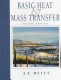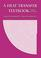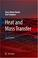### Mass and Heat Transfer

#### Course Description

Heat sources. Heat conduction in solid materials with and without internal heat sources. Basics of hydrodynamics. Conservation laws for fluid mass and momentum. Mass transfer. Convective heat transfer. Determination of heat transfer coefficients for forced and natural circulation. Heat transfer during phase change (boiling and condensation). Thermal radiation (radiation heat transfer). Heat exchangers and cooling towers. Numerical methods for heat and mass transfer (finite difference method, finite element method, finite volume method). Cooling of electric and electronic devices. Gas-vapor mixtures and air-conditioning.

#### General Competencies

Understanding of basic mass and heat transfer processes (conduction, convection, radiation). Ability to perform engineering and numerical calculations related to heat and mass transfer. Application of knowledge to the case studies related to: components and systems at power generating facilities; cooling of electric and electronic devices and energy efficiency.

#### Learning Outcomes

1. define the heat transfer types
2. explain mass, energy and momentum conservation laws
3. compute heat conduction within the solid plate, heat convection between the solid plate and fluid, and radiation heat exchange between two bodies
4. analyze the influence of variation in fluid physical parameters on heat transfer conditions
5. analyze the influence of plate geometry on heat transfer conditions
6. develop multidimensional heat transfer and fluid flow mathematical models
7. combine heat and mass transfer processes in power plant coolant systems
8. compare the efficiency of design solutions of the power facility components

#### Forms of Teaching

Lectures

Teaching the course is organized in two teaching cycles. The first cycle contains seven weeks, mid-term exam, and the second cycle contains six weeks of classes and a final exam. Classes are conducted through a total of 15 weeks with weekly load of 2 hours. Each lecture includes wrriten material and slides.

Exercises

Solved examples to support lectures and prepare students for exams. 1 hour per week. Solved examples on slides and discussion of possible variations in problem statement with examples on board.

Seminars

Application of computer codes for calculations of temperature distribution inside solid materials and velocity and pressure profiles during the flow of fluids.

By decision of the Faculty Council, in the academic year 2019/2020. the midterm exams are cancelled and the points assigned to that component are transferred to the final exam, unless the teachers have reassigned the points and the grading components differently. See the news for each course for information on knowledge rating.

Continuous Assessment Exam
Homeworks 0 % 15 % 0 % 15 %
Mid Term Exam: Written 0 % 30 % 0 %
Final Exam: Written 0 % 45 %
Final Exam: Oral 10 %
Exam: Written 0 % 75 %
Exam: Oral 10 %

#### Week by Week Schedule

1. Introduction. The different types of heat transfer (heat conduction, heat convection, radiative exchange). Main concepts and definitions. Technical applications.
2. Heat balance. Fourier’s law of heat conduction. Heat conductivity coefficient. Steady-state heat conduction in solid materials without the internal heat source.
3. Solid materials with internal heat sources. Heat conduction analysis for a nuclear reactor fuel rod.
4. Single phase fluid flow. Conservation laws for fluid mass and momentum. Mass transfer. Navier-Stokes equation. Bernoulli equation.
5. Fluid flow in pipes and channels. Laminar and turbulent flow. Theory of similarity of fluid flows. Dimensional analysis. Pressure drop for a single phase and a two phase flow.
6. Convective heat transfer. Experimental correlations for determination of the heat transfer coefficient. Heat transfer during forced flow and natural circulation.
7. Heat transfer in condensation and boiling. Subcooled and saturated nucleate boiling. Film boiling. Critical heat flux. The Nukiyama curve.
8. Exams.
9. Exams.
10. Radiation heat transfer. Black-body radiation – Stefan–Boltzmann law. Radiation in an enclosure. Emissivity and view factor.
11. Heat exchangers. Types of heat exchangers – cocurrent and countercurrent heat exchangers. The total heat transfer coefficient between fluids.
12. Mathematical analysis of heat exchangers. Logarithmic mean temperature difference. Heat exchangers efficiency.
13. Gas-vapor mixtures. Characteristic state variables. The psychrometric chart. Air-conditioning processes. Cooling towers.
14. Numerical methods (finite difference method, finite element method, finite volume method).
15. Application of numerical methods for the calculation of heat loads, mass transfer and fluid dynamics.

#### Study Programmes

Electrical Power Engineering (profile)
Specialization Course (2. semester)

#### Literature

Feretić, D. (2010.), Uvod u nuklearnu energetiku, 2. dopunjeno izdanje, Školska knjiga, ZagrebMills, A.F. (1999.), Basic Heat and Mass Transfer (2nd Edition), Prentice HallLienhard, J.H. IV, Lienhard, J.H. V (2003.), A Heat Transfer Textbook (3rd Edition), Phlogiston PressBaehr, H.D., Stephan, K. (2006.), Heat and Mass Transfer (2nd Edition), Springer
(.), A Heat Transfer Textbook J. H. Lienhard, J.H. Lienhard Phlogiston Press 2002,

#### General

ID 86506
Summer semester
4 ECTS
L1 English Level
L1 e-Learning
30 Lectures
15 Exercises
0 Laboratory exercises
0 Project laboratory